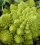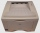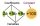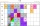# Fractions + third power - math problems

#### Number of problems found: 8

• MohammedMohammed grew a garden last year. His plot of land was 6 3/4 feet by 10 feet. He wants to have twice as much area and his garden this year. How much area will the new garden take up?
• Fraction unknownsDivide of fractions with unknowns: Fraction 1: The quantity x squared plus 6 times x plus 9 over the quantity x minus 1. Fraction 2: the quantity x squared minus 9 over the quantity x squared minus 2 times x plus 1.  Find Fraction 1 over Fraction 2.
• Two xeroxesThe performances of the two copiers are in the ratio 3: 4. A machine with higher power will make 7,200 copies in one hour. How many copies will both machines make together in 5 hours?
• Simplest formFind the simplest form of the following expression: 3 to the 2nd power - 1/4 to the 2nd power.
• Three machinesThe power of the three machines is 2: 3: 5. Two most powerful machines produce 400 parts per hour. How many components make all three machines in 3 hours?
• A tileA tile setter is covering 5ft by 5ft square shower wall. Each tile covers 4 5/8in by 4 5/8in square. How many rows of tile are needed to reach 5ft? How many tiles are needed to cover 5ft by 5ft square
• SeawaterSeawater has a density of 1025 kg/m3, ice 920 kg/m3. 8 liters of seawater froze and created a cube. Calculate the size of the cube edge.
• BMI indexCalculate BMI (body mass index, an index indicating obesity, overweight, normal weight, underweight) man weighing m = 71 kg and height h = 170 cm. The index is calculated according to equation (formula): BMI = (m)/(h2) With BMI index is possible to compa

We apologize, but in this category are not a lot of examples.
Do you have an exciting math question or word problem that you can't solve? Ask a question or post a math problem, and we can try to solve it.

We will send a solution to your e-mail address. Solved examples are also published here. Please enter the e-mail correctly and check whether you don't have a full mailbox.

Need help to calculate sum, simplify or multiply fractions? Try our fraction calculator. Fraction Word Problems. Third power - math problems.